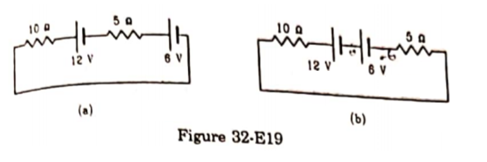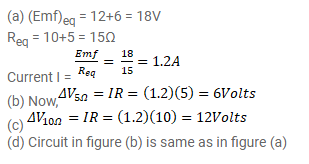# Consider the circuits shown in (32-E19a).

Question:

Consider the circuits shown in (32-E19a). Find

(a) The current in the circuit,

(b) The potential drop across the $5 \Omega$ resistor,

(c) The potential drop across the $10 \Omega$ resistor.

(d) Answer the parts (a), (b) and (c) with reference to figure (32-E19b).Solution: